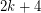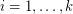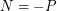# (3,6)-polyhedron

## Fowler's Conjecture on eigenvalues of (3,6)-polyhedra ★★

Author(s): Fowler

Conjecture   Letbe the graph of a-polyhedron withvertices. Then the eigenvalues ofcan be partitioned into three classes:,(whereis nonnegative for), and.

Keywords: (3,6)-polyhedron; eigenvalues